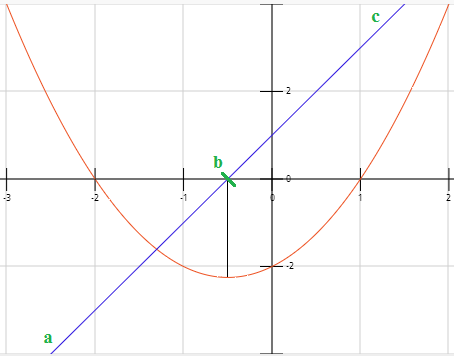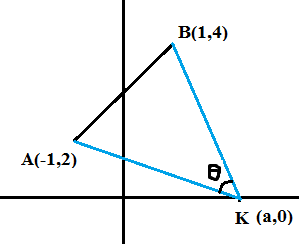# Slope of a polynomial

Slope is the angle made by a line with x - axis.  We usually say that slope = $Tan{\rm{ }}\theta$.
To find the slope of a linear equation we use the formula = $\displaystyle\frac{{{y_2} - {y_1}}}{{{x_2} - {x_1}}}$The graph drawn here is represented by y = 2x-1.  We consider y as a function of x.  So y = f(x) = 2x - 1.
So at point 'a' or x = 0  value of y = -1 and at point 'b' or x = 2 value of y = 3.
So slope is defined as the rate in change in y values over change in x value.
Slope = $\displaystyle\frac{{f(b) - f(a)}}{{b - a}}$ = $\displaystyle\frac{{3 - ( - 1)}}{{2 - 0}}$ = 2

But actual way of finding slope of a graph is the ratio of differences of f(x) at two different points on x- axis. say x+h, x where h is almost 0.  This is represented algebrically, $\begin{array}{*{20}{c}} {Lt}\\ {h \to 0} \end{array}\displaystyle\frac{{f(x + h) - f(x)}}{h}$

This is nothing but differentiation.

So $\begin{array}{*{20}{c}}{Lt}\\{h \to 0}\end{array}\displaystyle\frac{{2(x + h) - 1 - (2x - 1)}}{h} = \frac{{2h}}{h} = 2$

So if we take the graph of the slopes at various points on the function f(x) = 2x - 1 it is always 2.

Consider the following graph f(x) = ${x^2} + x - 2$. For this function slope cannot be determined uniquely as it changes across the line.  So we can only find slope at a given point x.   The blue line represents the differentiation of the given quadratic equation. It is represented by ${f^1}(x)$ = 2x + 1We also try to understand the relationship between the graph of the function and the graph of the differentiated function.

You can observe that the quadratic function attains a minimum value when the line of slope (blue line) touches the x- axis.  You can also observe, that the slope of the quadratic function is negative till it becomes zero (observe the blue line ab), and is positive (line bc) after that point.

We know that ${f^1}(x)$ = 2x + 1 and by equating this function to 0,  we get x = -1/2.  At this point the quadratic function attains its minimum value.

Consider the graphs of  $f(x) = {x^3} - 2{x^2} - x + 2$ and its differentiation ${f^1}(x) = 3{x^2} - 4x - 1$

You can observe that the slopes of the equation becomes zero two times.  These are called local maxima and minima.  This happens at points a and c.  But we know that a and c are nothing but the roots of the quadratic equation which inturns ${f^1}(x)$ which are $\displaystyle\frac{{1 \pm \sqrt 7 }}{3}$
What about point b.  Why the slope curve changed direction? this point is called inflection.  At this point, the function negative slope is maximum.  After this point, it is still decreasing, but not at the rate it used to be.That is why slope is still negative.

Concept Problems:

1. If ${x^4} - 14{x^2} + 24x - k = 0$ has four real and unequal roots, find the range where k lies.
If this equation has has to be 4 real roots, it must have 2 minima, both must happen below the x - axis and and 1 maxima, to be happen above x - axis.  Then only the graph cuts the x-axis at 4 points.
Now, To find where this local maxima and minimas happen, we differentiate f(x) and equate to zero.
${f^1}(x) = 4{x^3} - 28x + 24$ = 4(x-1)(x-2)(x+3)

So ${f^1}(x)$ has the roots -3, 1, 2.
Now f(2) = 16 - 56 + 48 - k = 8 - k
and f(1) =  1 -14 + 24 - k = 11 - k
and f(-3) = 81 - 126 -72 -k = -117 - kWe know that f(x) is an increasing function. So it attains minima firstly at 2.  So 8 - k < 0.  and attains maxima at 1. So 11 - k > 0. and again attains minima at - 3. so -117 - k < 0.  Solving above equations we get k > 8, k > -117, and k < 11We have to get the region which satisfies all the three equations. So k must lie in between 8 and 11.

2.  A ≡ (–1, 2), B ≡ (1, 4). Point K lies on the x-axis. What is the area of ΔAKB when ∠AKB is maximum, with K on the positive x-axis?
1)16 sq. units  2) 6 sq. units 3) 4 sq. units 4) Cannot be determined
Sol:Slope of the line AK = $\displaystyle\frac{{{y_2} - {y_1}}}{{{x_2} - {x_1}}}$ = $\displaystyle\frac{2}{{ - 1 - a}}$
Slope of the line BK = $\displaystyle\frac{4}{{1 - a}}$

Let us find the angle between the lines using slopes. We know that $Tan\theta = \displaystyle\frac{{{m_1} - {m_2}}}{{1 + {m_1}{m_2}}}$
$\Rightarrow Tan\theta = \displaystyle\frac{{\displaystyle\frac{{ - 2}}{{1 + a}} - \displaystyle\frac{4}{{1 - a}}}}{{1 + \displaystyle\frac{{ - 8}}{{1 - {a^2}}}}}$
$\Rightarrow \displaystyle\frac{{\displaystyle\frac{{ - 2 + 2a - 4 - 4a}}{{1 - {a^2}}}}}{{\displaystyle\frac{{1 - {a^2} - 8}}{{1 - {a^2}}}}} = \displaystyle\frac{{ - 2a - 6}}{{ - {a^2} - 7}} = \displaystyle\frac{{2a + 6}}{{{a^2} + 7}}$

We need to maximum $\theta$ so we have to differentiate the given function w.r.t to independent variable 'a'

$Se{c^2}\theta .\displaystyle\frac{{d\theta }}{{da}} = \displaystyle\frac{{({a^2} + 7)2 - 2a(2a + 6)}}{{{{\left( {{a^2} + 7} \right)}^2}}}$

To find the maxima, we should equate this function to '0'
So we get $2{a^2} + 14 - 4{a^2} - 12a$ = 0
Or, ${a^2} + 6a - 7$
Or a = 1 or - 7.

Given K is on the x - axis. So a = 1
We have to now find Area of the triangle with co-ordinates (-1, 2), (1, 4), and (1,0)
Add (-1, 0) to all coordinates = (-2, 2), (0, 4), (0,0)

The area of the triangle whose coordinates are (0,0), $\left( {{x_{1,}}{y_1}} \right)$, $\left( {{x_{2,}}{y_2}} \right)$ is $\displaystyle\frac{1}{2}\left| {\left[ {{x_1}.{y_2} - {x_2}.{y_1}} \right]} \right|$

So By applying above formula we get area of the triangle = 4 units.